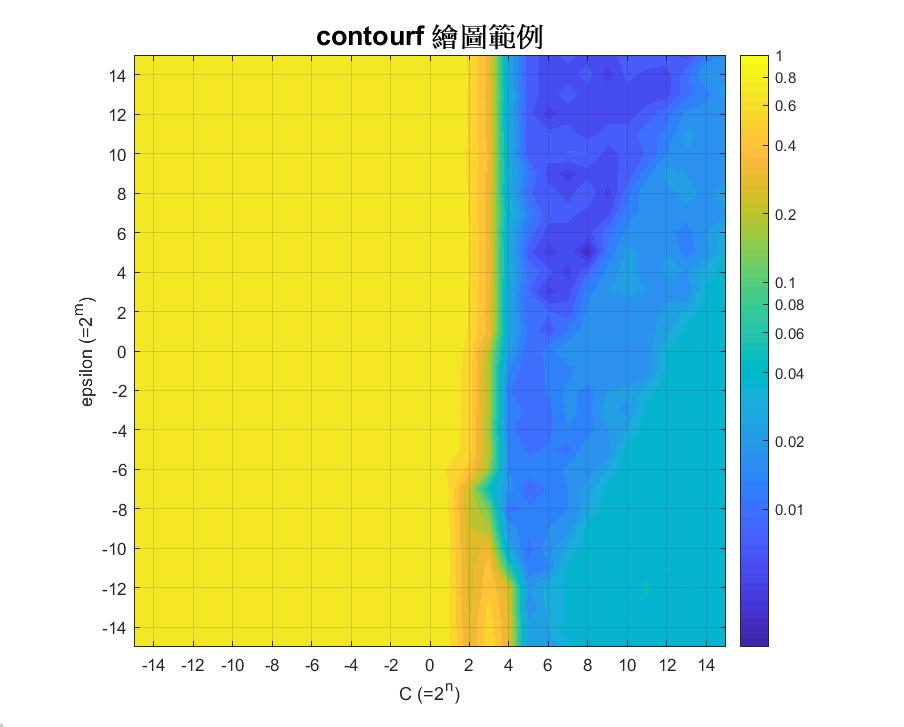### 【MATLAB】contourf 基礎用法與對數作圖

• 848
• 0
• 2019-10-16

MATLAB      Version 9.6      (R2019a)

load('data.mat');

## 一. 基礎用法

subplot(121)
contour(data)
title('contour function') % 小標-1

subplot(122)
contourf(data)
title('contourf function') % 小標-2

% 大標題
h = sgtitle('contour vs contourf');
h.FontSize = 16;
h.FontWeight = 'bold';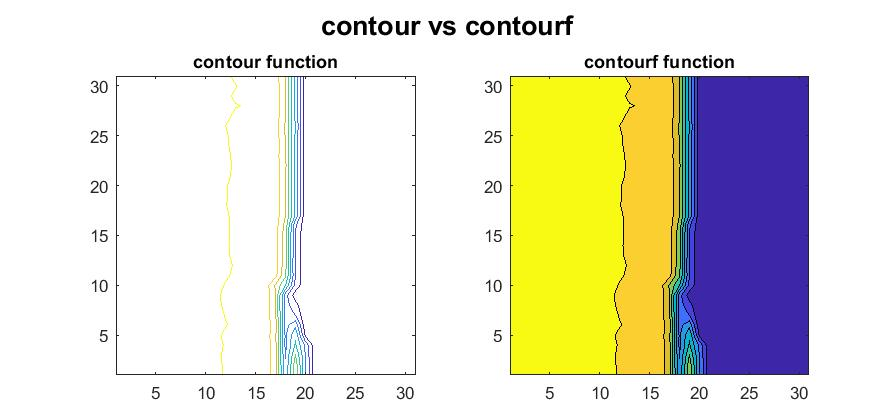#### 1. 等高線數量

subplot(121)
contourf(data, 1)
title('1 contour line')

subplot(122)
contourf(data, 5)
title('5 contour lines')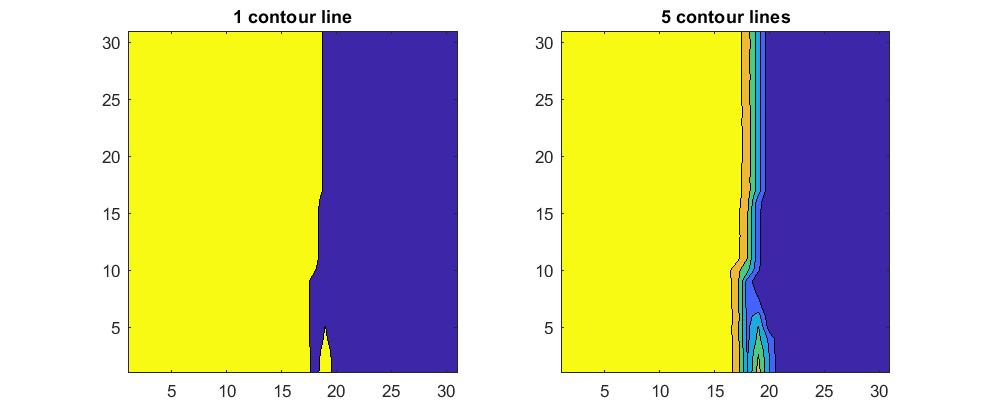#### 2. 等高線粗細 & 型態

subplot(221)
contourf(data, 3, 'LineWidth', 2)
title('LineWidth = 2')

subplot(222)
contourf(data, 3, 'LineStyle', '--')
title('LineStyle = "--"')

% 不要繪製等高線也可以
subplot(224)
contourf(data, 3, 'LineStyle', 'none')
title('LineStyle = "none"')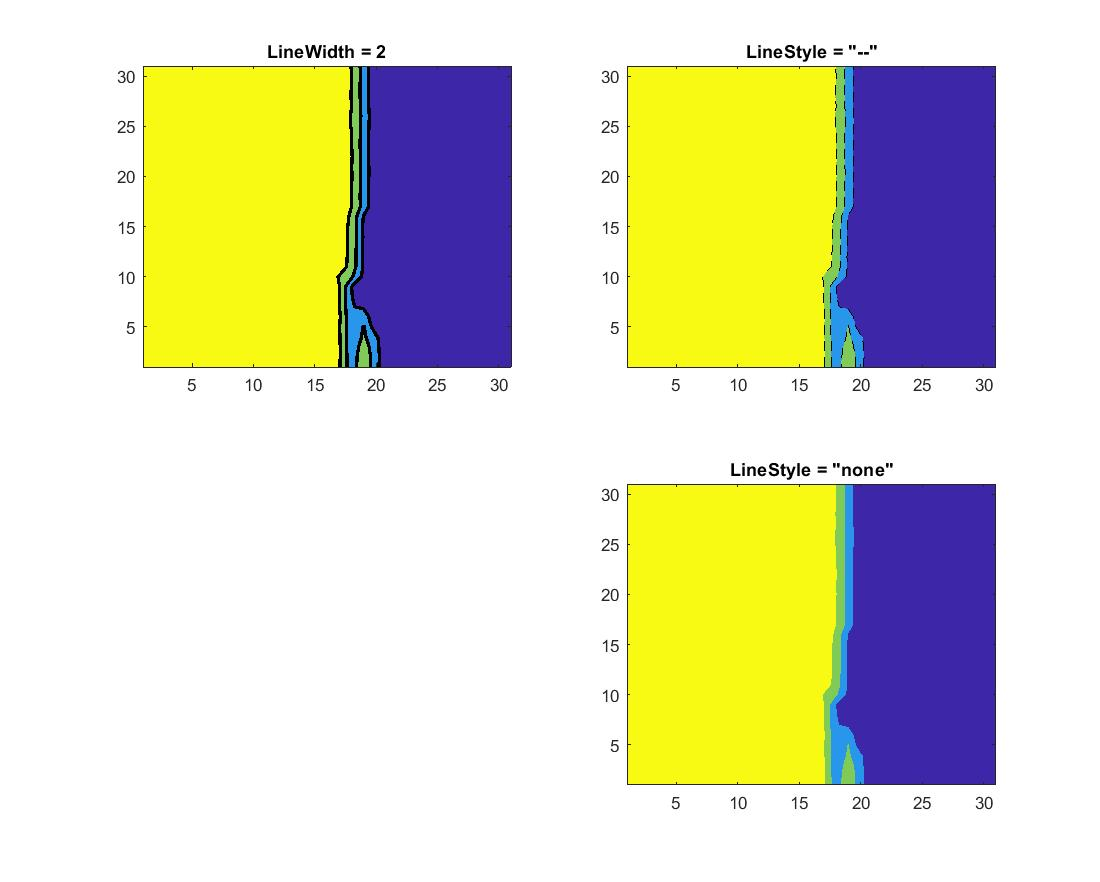#### 3. 配色盤(colormap)

% colormap('map') or colormap map

contourf(data, 10)
colormap gray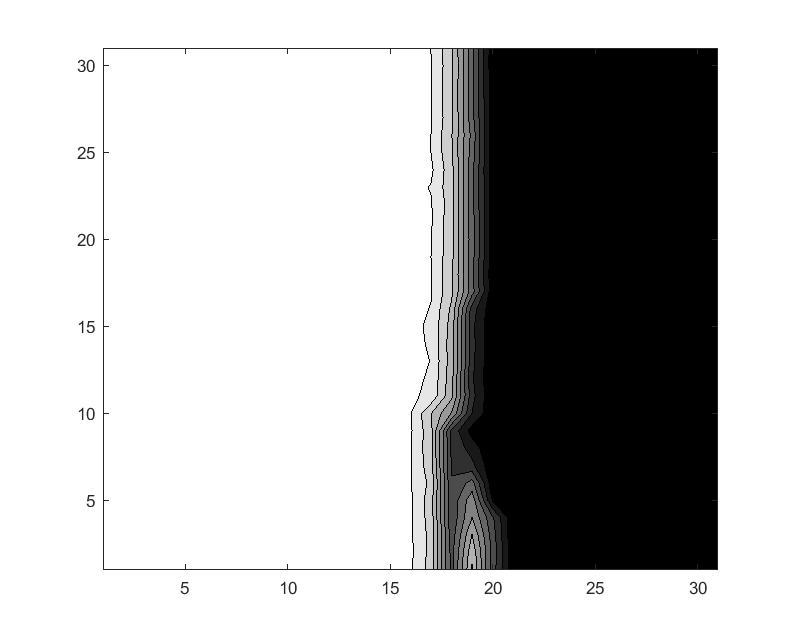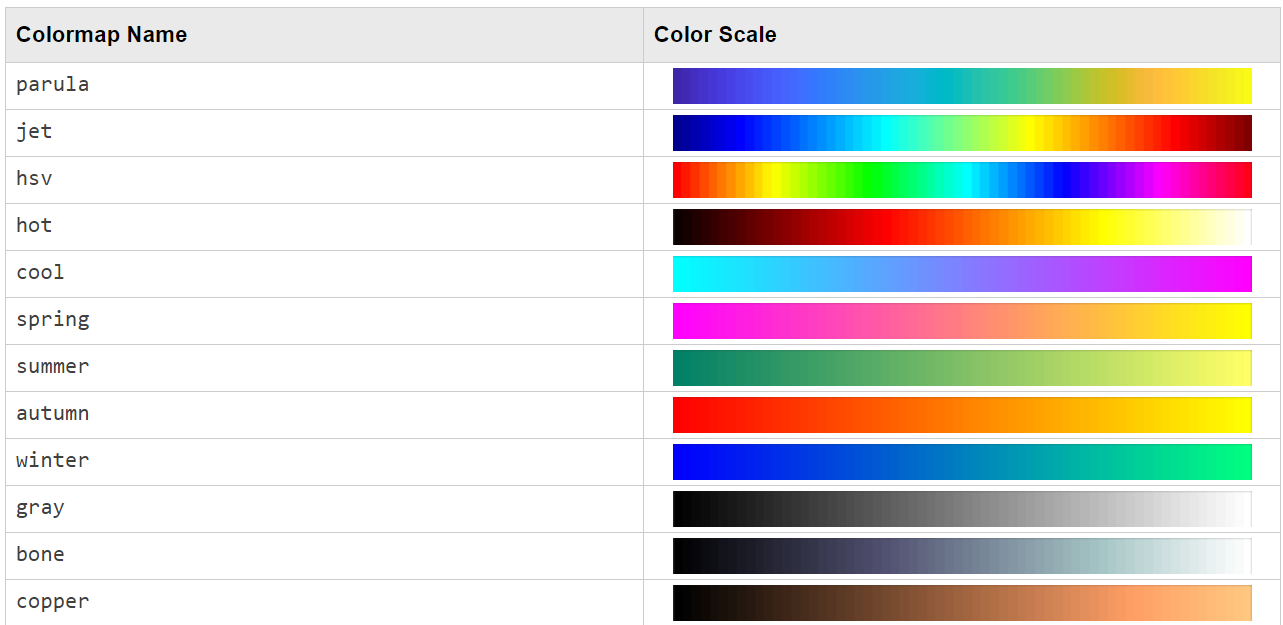配色盤圖片擷取自 MATLAB 說明文件

% contour levels
levels = 4;

contourf(data, levels)
colormap(jet(levels+1))
colorbar
title('color = levels+1')

contourf(data, levels)
colormap(jet(levels+10))
colorbar
title('color = levels+10')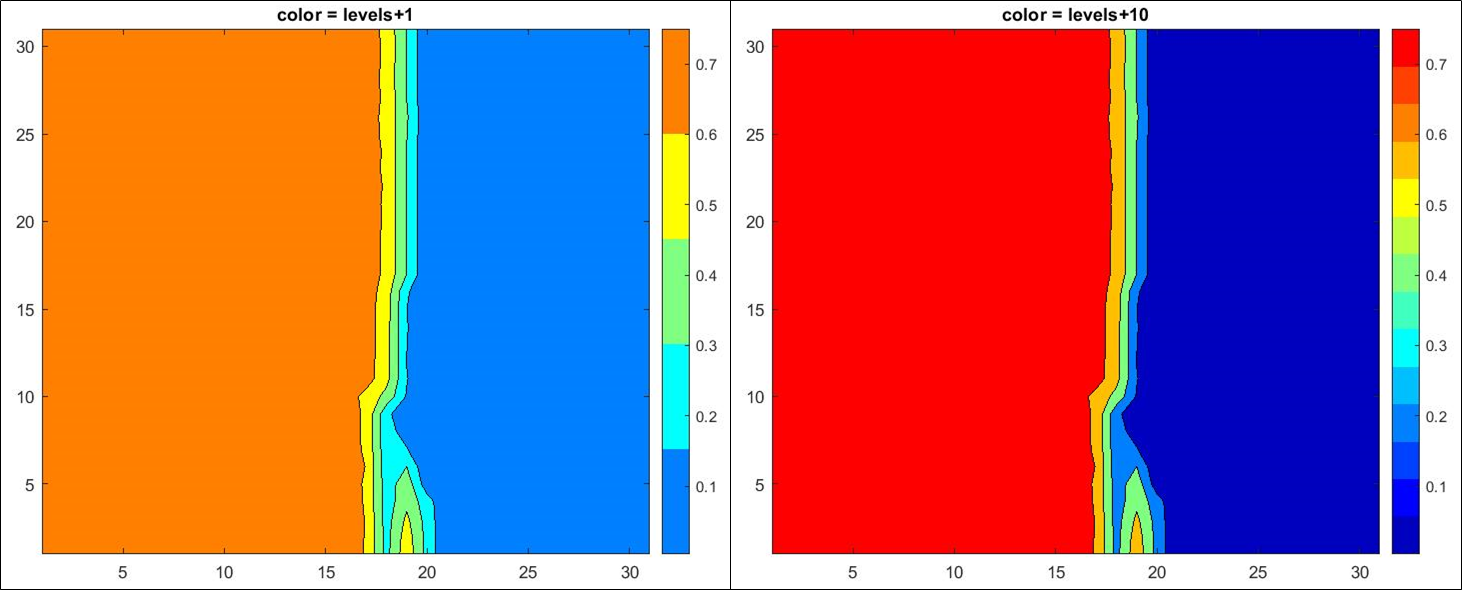在 subplot 中使用 colormap，會將所有子圖全部套用相同的調色盤。

#### 4. 設定資料邊界

caxis 的用法是：caxis( [ 下界 , 上界 ] )，若在此界線之外，則全部配給同一顏色。

contourf(data, 10)

% 低於 0.3 只給一種顏色
% 高於 0.7 亦只給一種顏色
caxis([0.3 0.7])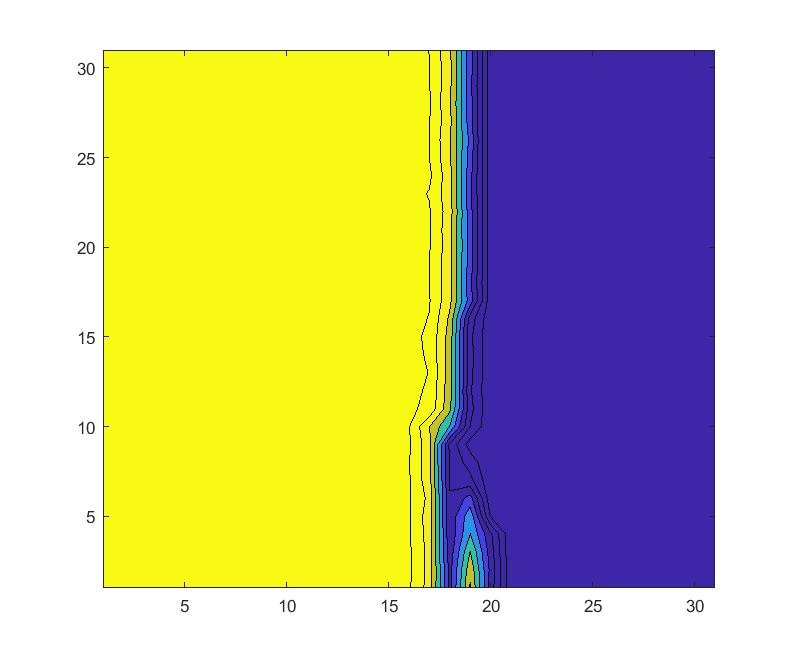#### 5. 修改座標軸範圍

% contour levels
levels = 10;

contourf(data, levels, 'LineStyle', 'none')
grid on

% x 座標軸修改
xticks(2:2:30)
xticklabels(gca, -14:2:14)

% y 座標軸修改
yticks(2:2:30)
yticklabels(gca, -14:2:14)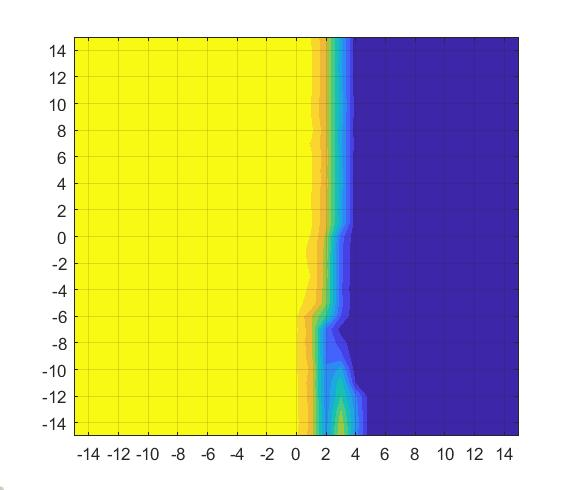## 二、進階應用

% Natural logarithm
ln_data = log(data);

subplot(121)
normplot(data(:))
title('original')

subplot(122)
normplot(ln_data(:))
title('log')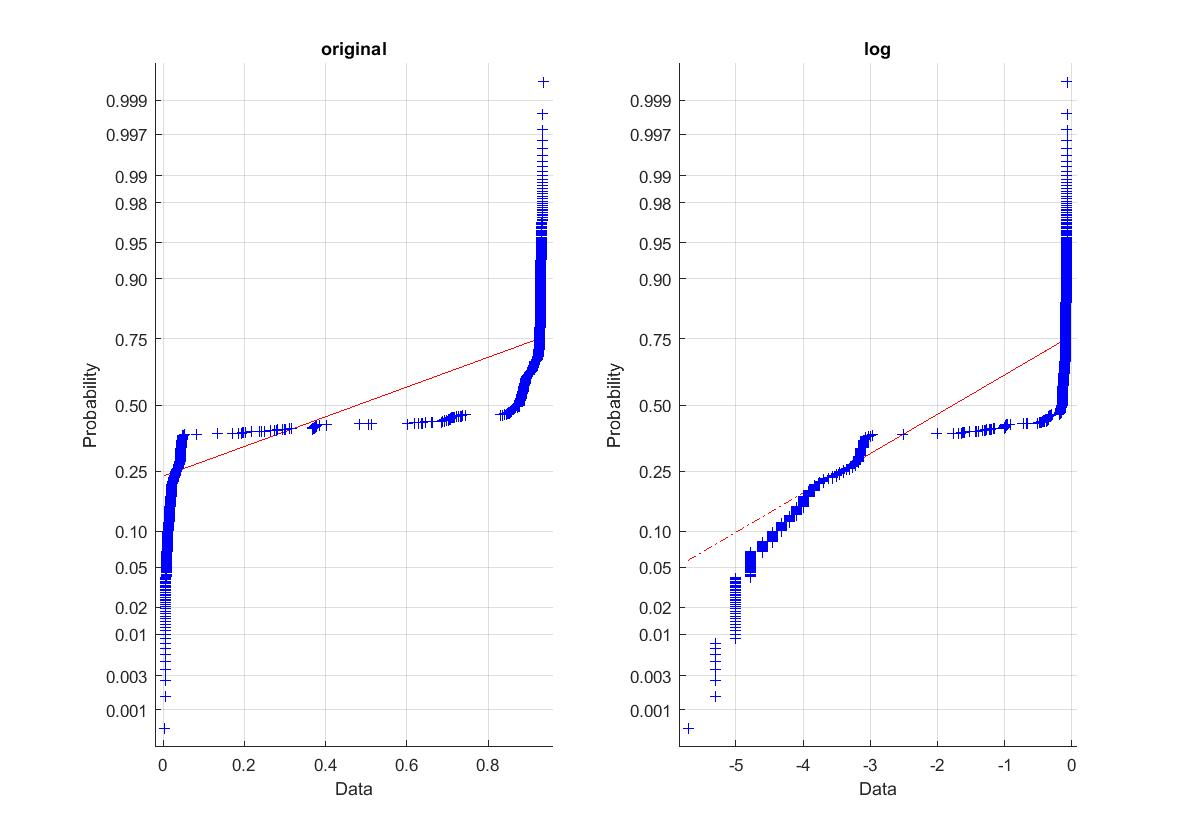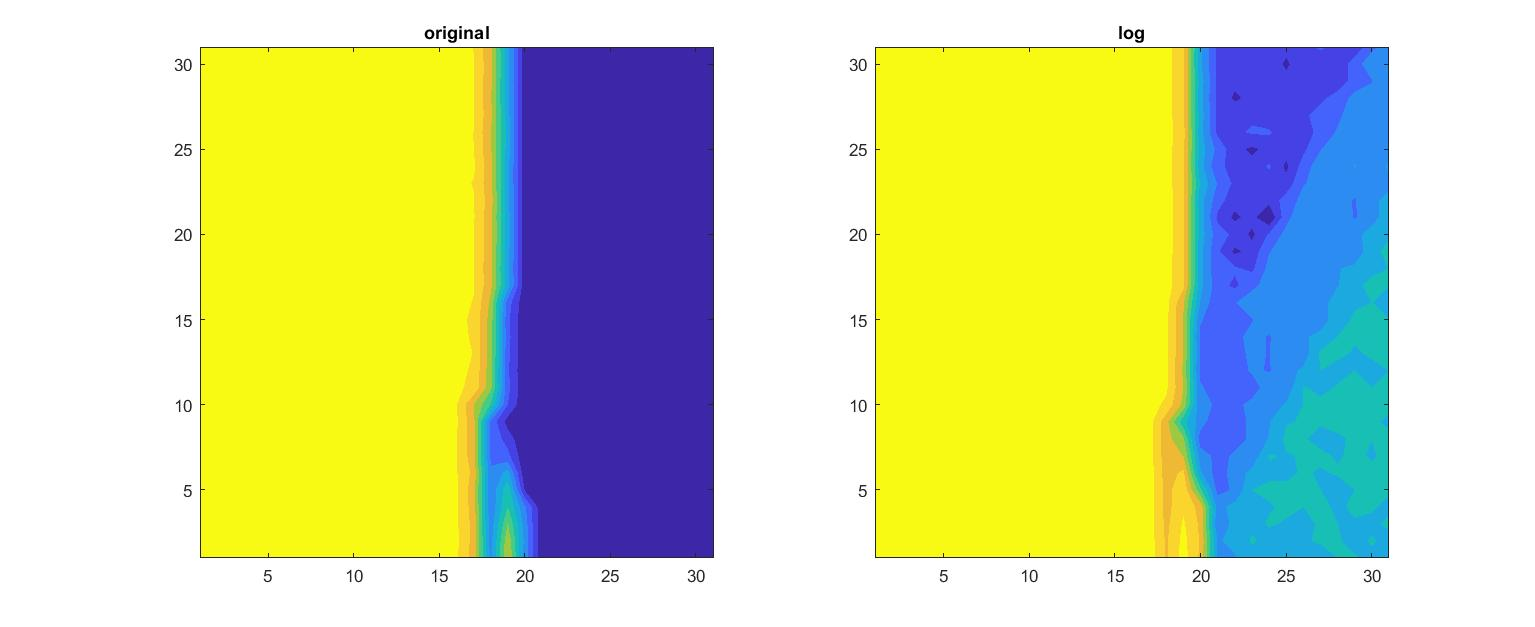% plot sigmoid function

% sigmoid 數學式為 y = 1/(1+e^(-x))
x = -10:0.01:10;
y = 1./(1+exp(-x));
plot(x, y, 'b ','LineWidth', 2)

% 當 X 等於零，其對應值為 0.5
hold on
plot([-10 10], [0.5 0.5], 'k--','LineWidth', 1)
hold off

% 限制圖表範圍，看起來較美觀
grid on
axis([-10 10 -0.1 1.1])

% 手動給定標籤
yticks([0 0.2 0.4 0.5 0.6 0.8 1])

% 使用 latex 語法，撰寫公式文字
text(-9.5, 0.9, 'S(t) =', 'interpreter','latex' , 'FontSize', 14);
text(-7, 0.9, '$\frac{1}{1+e^{-x}}$', 'interpreter','latex', 'FontSize', 24);

% 給一個好聽的標題名稱
title('Sigmoid function')#### 1. 多圖比較

% 設定資料變動範圍
min_boundary = floor(log(min(data(:))));
caxis( [ min_boundary , 0 ] )

% Natural logarithm
ln_data = log(data);
% contour levels
levels = 10;

% ======== 圖1 ======== %
subplot(121)
contourf(data, levels, 'LineStyle', 'none')
title('original')
grid on

% 座標修改
xticks(2:2:30)
xticklabels(gca, -14:2:14)
yticks(2:2:30)
yticklabels(gca, -14:2:14)

% 邊界設定
caxis( [ -6 , 1 ] )
colorbar

% ======== 圖2 ======== %
subplot(122)
contourf(ln_data, levels, 'LineStyle', 'none')

title('log')
grid on

% 座標修改
xticks(2:2:30)
xticklabels(gca, -14:2:14)
yticks(2:2:30)
yticklabels(gca, -14:2:14)

% 邊界設定
caxis( [ -6 , 1 ] )
colorbar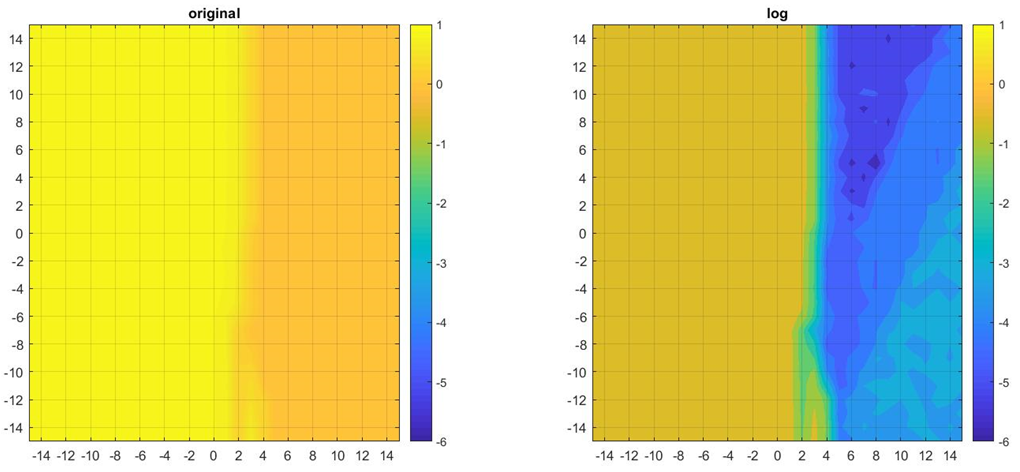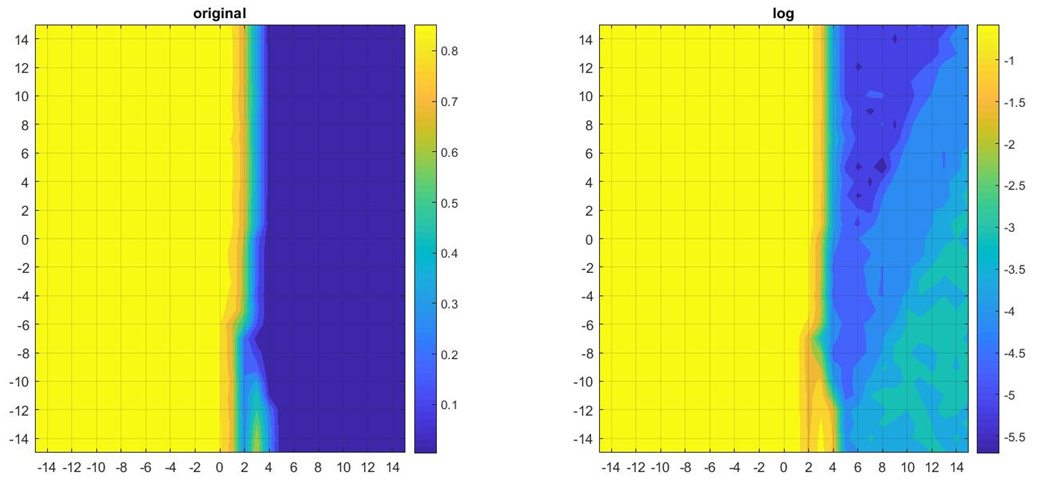#### 2. 一般的 colorbar 調值

% contour levels
levels = 10;

subplot(121)
contourf(data, levels, 'LineStyle', 'none')

% 指定 colorbar 數值
c = 0:0.1:1;
colorbar('YTick', c)
title('colorbar 間隔=0.1')

subplot(122)
contourf(data, levels, 'LineStyle', 'none')

% 指定 colorbar 數值
c = 0:0.2:1;
colorbar('YTick', c)
title('colorbar 間隔=0.2')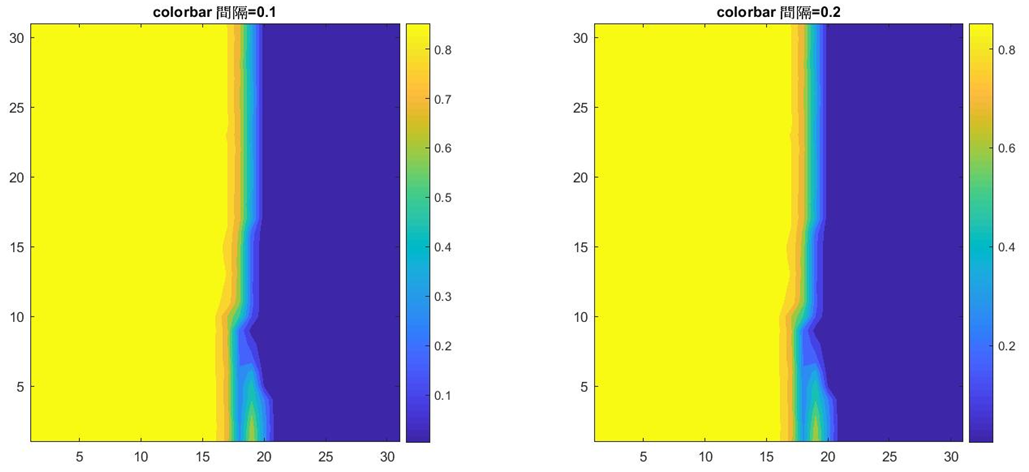% contour levels
levels = 10;

contourf(data, levels, 'LineStyle', 'none')

c = 0:0.1:1;
c_label = 0:10:100;

colorbar('YTick', c, 'YTickLabel', c_label)
title('colorbar 修改 YTickLabel')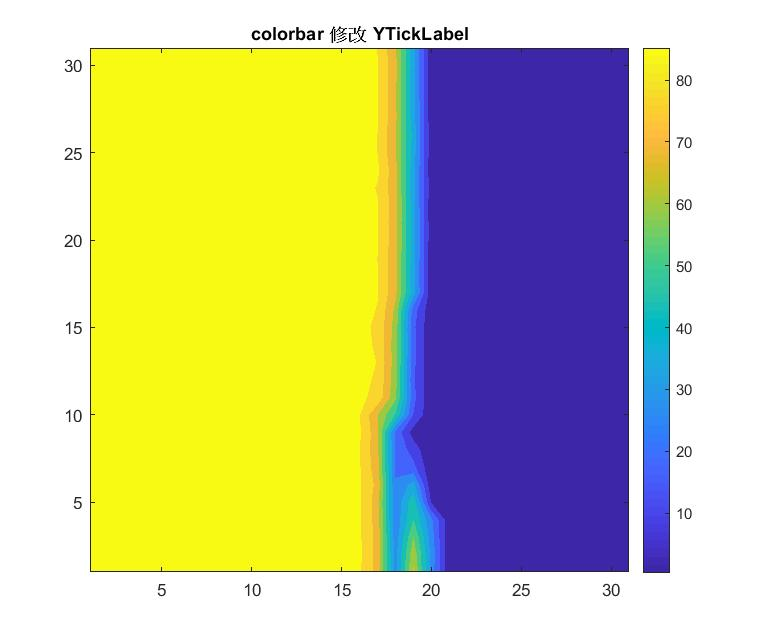原因是沒有使用 caxis 限定，因此會透過資料數值自動計算上下界。

#### 3. 對數轉換後的 colorbar 調值

% Natural logarithm
ln_data = log(data);
% contour levels
levels = 10;

contourf(ln_data, levels, 'LineStyle', 'none')

% 邊界設定
min_boundary = floor(log(min(data(:))));
caxis( [ min_boundary , 0 ] )

% colorbar 數值設定
c = log( [0.01, 0.02:0.02:0.1, 0.2:0.2:1] );
c_label = [0.01, 0.02:0.02:0.1, 0.2:0.2:1];

colorbar('YTick', c, 'YTickLabel', c_label)
title('對數轉換時 colorbar 修改')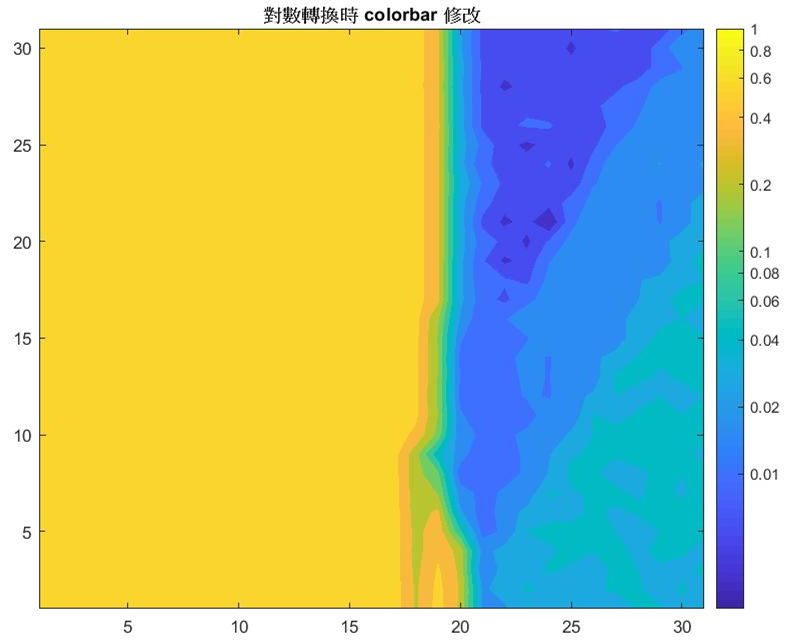## 小結

% Plot contour figure
%
% 2019.09.04 Shayne

% Natural logarithm
ln_data = log(data);

% contour levels
levels = 20;

contourf(ln_data, levels, 'LineStyle', 'none')
axis equal
grid on

% 座標修改
xticks(2:2:30)
xticklabels(gca, -14:2:14)
yticks(2:2:30)
yticklabels(gca, -14:2:14)

% 邊界設定
min_boundary = floor(log(min(data(:))));
caxis( [ min_boundary , 0 ] )

% colorbar 數值設定
c = log( [0.01, 0.02:0.02:0.1, 0.2:0.2:1] );
c_label = [0.01, 0.02:0.02:0.1, 0.2:0.2:1];
colorbar('YTick', c, 'YTickLabel', c_label)

% 標題 & 座標軸
title('contourf 繪圖範例', 'FontSize', 16)
xlabel('C (=2^n)')
ylabel('epsilon (=2^m)')